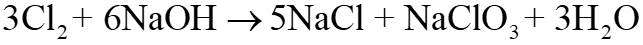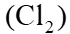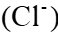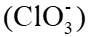Assertion (A): Chlorine gas disproportionate in hot and conc. NaOH solution. Reason (R): NaCl and NaOCl and are formed in the above reaction.

# Assertion (A): Chlorine gas disproportionate in hot and conc. solution. Reason (R): and and are formed in the above reaction.

1. A
Both (A) and (R) are true and (R) is the correct explanation of (A).
2. B
Both (A) and (R) are true but (R) is not the correct explanation of (A).
3. C
(A) is true but (R) is false.
4. D
(A) is false and (R) is true.

Fill Out the Form for Expert Academic Guidance!l

+91

Live ClassesBooksTest SeriesSelf Learning

Verify OTP Code (required)

### Solution:

Concept: In redox chemistry, the disproportion reaction is the process by which one chemical with an intermediate oxidation state splits into two compounds, one of which gains a higher oxidation state and the other a lower one.
When concentrated NaOH react with Chlorine:The chlorine oxidation state in the reactantis 0 and in products is: of chloride ion is -1and that of chlorate ion is +5. Therefore, it's a disproportionate reaction. Thereby, the assertion given is true.
As we can see, the products of the reaction are not and . Thus, the reason is wrong.
Hence, the correct answer is option 3.

## Related content

 Is Rajju Bhaiya University Recognized by The University Grants Commission? Golden Opportunity with Sri Chaitanya’s Score Scholarship Exam 2023 100 Paryayvachi Shabd (पर्यायवाची शब्द) – Paryayvachi Shabd in Hindi (Synonyms) Watts to horsepower conversion Infinity Learn: Rankguru Technology Solutions Private Limited BOD Full Form: Biochemical Oxygen Demand GMT Full Form: Greenwich Mean Time Manure and fertilisers Kidney Volume of a Pyramid Formula+91

Live ClassesBooksTest SeriesSelf Learning

Verify OTP Code (required)